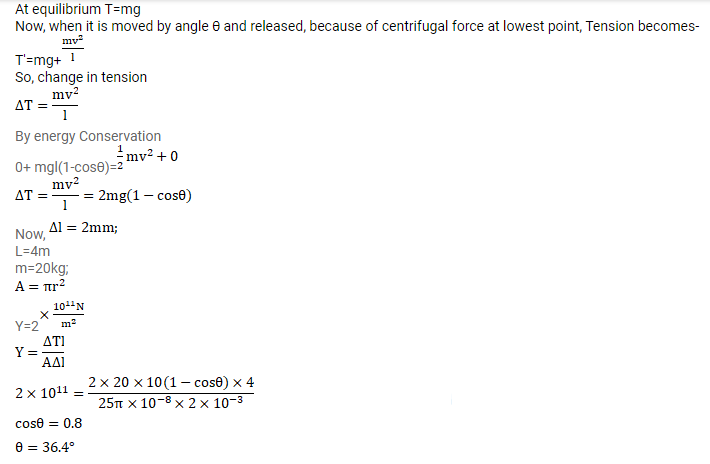# A sphere of mass 20 kg is suspended by a metal wire ofQuestion:

A sphere of mass $20 \mathrm{~kg}$ is suspended by a metal wire of unstretched length $4 \mathrm{~m}$ and diameter $1 \mathrm{~mm}$. When in equilibrium, there is a clear gap of $2 \mathrm{~mm}$ between the sphere and the floor. The sphere is gently pushed aside so that the wire makes an angle $\theta$ with the vertical and is released. Find the maximum value of $\theta$ so that the sphere does not rub the floor. Young's modulus of the metal of the wire $2.0 \times 10^{11} \mathrm{~N} / \mathrm{m}^{2}$. Make appropriate approximations.

Solution: